Courses

# Doc: Electrical Properties (Conductors, Insulators and Semiconductors) Notes | EduRev

## Class 12 : Doc: Electrical Properties (Conductors, Insulators and Semiconductors) Notes | EduRev

The document Doc: Electrical Properties (Conductors, Insulators and Semiconductors) Notes | EduRev is a part of the Class 12 Course Chemistry Class 12.
All you need of Class 12 at this link: Class 12

Electrical Conductivity:

• The electrical conductivity of solids is due to the motion of electrons or positive holes. The conductivity due to the motion of electron or positive holes is called electronic conductivity. The electrical conductivity may be due to the motion of ions. The conductivity due to the motion of ions is called ionic conductivity. Conductivity due to electrons is called n-type conductivity while that due to holes is called p-type conductivity.
• In metals, electrical conductivity is due to the motion of electrons and the electrical conductivity increases with the increase in the electrons available for conduction, the electrical conductivity increases.
• Pure ionic solids ions are not available for conduction hence in the pure solid state they are insulators. Due to the presence of defects in the crystal electrical conductivity increases.
• Solids exhibit a varying range of electrical conductivities, extending of magnitude ranging from 10–20 to 107 ohm–1 m–1. Solids can be classified into three types on the basis of their conductivities. The difference in conductivities of conductors, insulators and semiconductors can be explained on the basis of band theory.

Electrical Conductivity on the Basis of Energy Bands:

• The group of discrete but closely spaced energy levels for the orbital electrons in a particular orbit is called energy band.
• Inside the crystal, each electron has a unique position and no two electrons see exactly the same pattern of surrounding charges. Because of this, each electron will have a different energy level. These different energy levels with continuous energy variation form what are called energy bands.
• The energy band which includes the energy levels of the valence electrons is called the valence band. The energy band above the valence band is called the conduction band. With no external energy, all the valence electrons will reside in the valence band.

Conductors:

• The solids with conductivities ranging between 104 to 107 ohm–1 m–1 are called conductors.
• Metals have conductivities in the order of 107 ohm–1 m–1 are good conductors.
• In conductors, the lowest level in the conduction band happens to be lower than the highest level of the valence band and hence the conduction band and the valence band overlap. Hence the electron in the valence band can migrate very easily into the conduction band. Thus at a room temperature, a large number of electrons are available for conduction.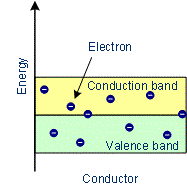Fig: Variation of energy in Conductors

Examples: Copper, Aluminium, Silver, Gold, All metals

Characteristics of Conductors:

• The substances which conduct electricity through them in greater extent are called conductors.
• In conductors, the conduction band and valence band overlap with each other or gap between them is very small.
• There are free electrons in the conduction band.
• Due to increase in temperature conductance decreases.
• There is no effect of the addition of impurities on the conductivity of conductors.
• Their conductivity ranges between 104 to 107 ohm–1 m–1.

Conduction in Metallic Solids:

• A metal conductor conducts electricity through movement of free electrons. Metals conduct electricity in solid as well as a molten state. The conduction of electricity is due to transfer of electrons and not due to the transfer of matter.
• The conductivity of metals depends upon the number of valence electrons available per atom. It is nearly independent of the presence of impurity and lattice defects.
• Conductivity decreases with the increase in the temperature. It can be explained as follows:

M → Mn+kernel + ne  free electrons
The kernels are fixed. Due to increase in temperature, the amplitude of vibration of kernels increases. Hence the obstruction to the flow of electrons increases.

Insulators:

• These are the solids with very low conductivities ranging between 10–20 to 10–10 ohm–1 m–1.
• The conduction band and valence band are widely spaced. Thus forbidden energy gap between the valence band and conduction band is large (greater than 3 eV). Hence the electron in the valence band cannot migrate into the conduction band. Hence no electrons are available for conduction. But at a higher temperature, some of the electrons from the valence band may gain external energy to cross the gap between the conduction band and the valence band. Then these electrons will move into the conduction band. At the same time, they will create vacant energy levels in the valence band where other valence electrons can move. Thus the process creates the possibility of conduction due to electrons in conduction band as well as due to vacancies in the valence band.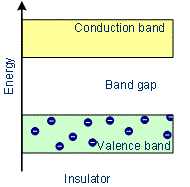Fig: Variation of energy in insulators

Examples: Glass, wood, paper, plastic, mica.

Characteristics of Insulators:

• In Insulators the conduction band and valence band are widely separated.
• There are no free electrons in the conduction band.
• There is an energy gap between the conduction band and valence band which is more than 3 eV.
• There is no effect of change of temperature on the conductivity of insulators.
• There is no effect of the addition of impurities on the conductivity of insulator.
• They have very low conductivities ranging between 10–20 to 10–10ohm–1m–1.

Semiconductors:

• These are the solids with conductivities in the intermediate range from 10–6 to 104 ohm–1 m–1.
• The forbidden energy gap between the valence band and conduction band is less than 3 eV. Thus energy gap between the valence band and conduction band is small. At absolute zero, no electrons are available for conduction.
• As the temperature increases, many electrons from the valence band may gain external energy to cross the gap between the conduction band and the valence band. Then these electrons will move into the conduction band. At the same time, they will create vacant energy levels in the valence band where other valence electrons can move. Thus the process creates the possibility of conduction due to electrons in the conduction band as well as due to vacancies in the valence band.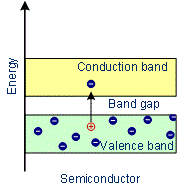Fig: Variation of energy in Semiconductors

Examples: Silicon, Germanium

Characteristics of Semiconductors:

• In semiconductors, the conduction band and valence band are very close to each other or the forbidden energy gap between them is very small.
• The electrons of valence bond can easily be excited to the conduction band.
• There is an energy gap between conduction band and valence band which is less than 3 eV.
• Due to increase in temperature conductance increases.
• There is an effect of the addition of impurities on the conductivity of semiconductors.
• Their conductivity ranges from 10–6 to 104 ohm–1 m–1.

Doping of Semiconductors:
n doping: This involves substituting Si by neighboring elements that contribute excess electrons. For example, small amounts of P or As can substitute Si.

Since P/As have 5 valence electrons, they behave like Si plus an extra electron. This extra electron contributes to electrical conductivity, and with a sufficiently

large number of such dopant atoms, the material can displays metallic conductivity. With smaller amounts, one has extrinsic n-type semiconduction.
Rather than n and p being equal, the n electrons from the donor usually totally outweigh the intrinsic n and p type carriers so that:
σ ∼n|e|μe
The donor levels created by substituting Si by P or As lie just below the bottom of the conduction band. Thermal energy is usually sufficient to promote the donor electrons into the conduction band.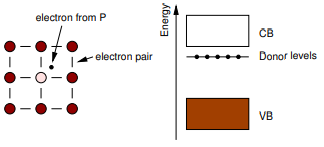Fig: N type doping

p doping: This involves substituting Si by neighboring atom that has one less electron than Si, for example, by B or Al. The substituent atom then creates a “hole” around it, that can hop from one site to another. The hopping of a hole in one direction corresponds to the hopping of an electron in the opposite direction. Once again, the dominant conduction process is because of the dopant.

σ ∼ρ|e|μh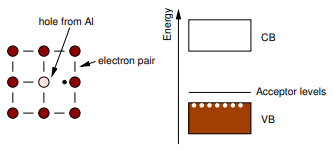Fig: P type doping

T dependence of the carrier concentration The expression: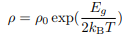can inverted and written in terms of the conductivity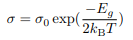Now σ = n|e|µe or σ = p|e|µh. It is known that the mobility µ is effectively temperature-independent so we can express the carrier concentration in terms
of temperature: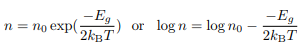for an electron-doped semiconductor and for a hole-doped semiconductor: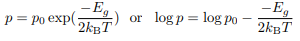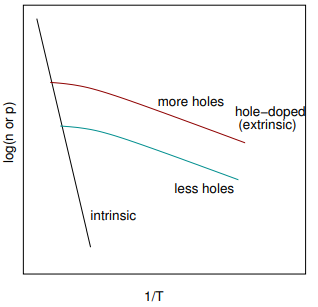The plot above shows typical variation of the logarithm of the carrier concentration with inverse temperature. At high temperatures (small 1/T) the data follows usual activated behavior of an intrinsic semiconductor. At lower temperatures (larger 1/T) extrinsic behavior dominates.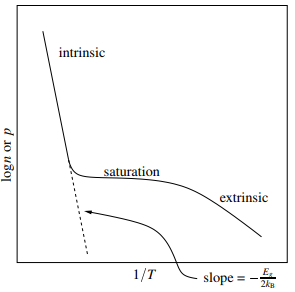Initially, lowering the temperature results in saturation of the acceptor levels or exhaustion of the donor levels. Only at still lower temperatures does the extrinsic behavior take over.

Semiconductor devices:
The p − n junction is formed when the two different sides of semiconductor are doped, respectively with holes (for example, Al for Si) and electrons
(for example, P for Si). One of the properties of the p − n junction is that it rectifies — it allows an electric current to pass only in one direction.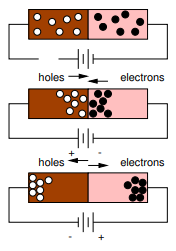Fig: Flow of electron in p-n junction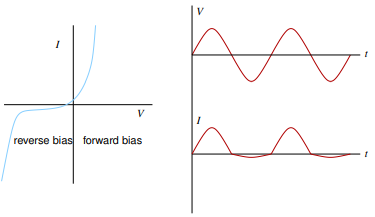Fig: V - I graph of p-n junction

The junction transistor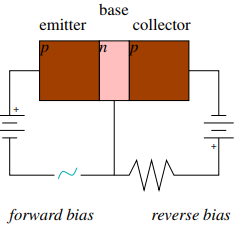Fig: PNP BJT with forward-biased p-n junction and reverse-biased n-p  junction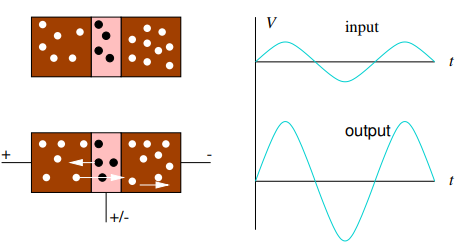Fig: a Flow of electrons in pnp junction
Fig: b V-I graphs of pnp  junction

Offer running on EduRev: Apply code STAYHOME200 to get INR 200 off on our premium plan EduRev Infinity!

,

,

,

,

,

,

,

,

,

,

,

,

,

,

,

,

,

,

,

,

,

,

,

,

;